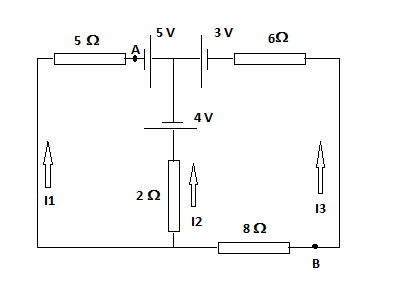# Given the following circuit and current directions. a. Find all the currents. State clearly the...

## Question:

Given the following circuit and current directions.

a. Find all the currents. State clearly the direction of your loop rule applied.

b. Determine {eq}V_{AB}. {/eq}## Kirchhoff's Rules

Kirchhoff's rules include the junction rule and the loop rule. The junction rule states stems from the conservation of electric charge. Current into a junction equals the current leaving the junction. The loop rule sums all voltages in a closed loop and sets the sum equal to zero. This rule stems from the law of the conservation of energy.

#### Part a

The current directions of the currents is impossible. The diagram shows all three currents entering a junction. Let's change current 2 to be moving downward in the diagram instead of upward. We'll write a junction rule equation first for the top middle junction.

{eq}I_1+I_3=I_2\\ I_1-I_2+I_3=0\\ {/eq}

Now we'll write an equation using the loop rule for the left branch of the circuit. We'll start at the bottom left corner of the circuit and move clockwise staying in the left loop.

{eq}-5I_1+5-4-2I_2=0\\ -5I_1-2I_2+0I_3=-1\\ {/eq}

Now we'll write an equation using the loop rule for the right branch of the circuit. We'll start at the bottom right corner of the circuit and move counterclockwise staying in the right loop.

{eq}-6I_3+3+4-2I_2-8I_3=0\\ 6I_1-2I_2-14I_3=-7\\ {/eq}

Now we'll plug these equations into a matrix calculator to determine the currents. We get: {eq}I_1=0.019\:amp\\ I_2=0.454\:amp\\ I_3=0.435\:amp\\ {/eq}

#### Part b

The voltage {eq}V_{AB} {/eq} is 8.09 V.

We'll use the loop rule starting at point A moving through the junction, through the 3 V battery and through the 6 Ω and 8 Ω resistors ending at point B. We don't set this equation equal to zero because it isn't a closed loop.

{eq}V_{AB}=5-3+(6)(0.435)+(8)(0.435)\\ V_{AB}=8.09\:\rm volts {/eq}